Contents | Prev | Next | Index

CHAPTER 21

# The Package java.util

The `java.util` package contains various utility classes and interfaces.

Notable among these utilities is the `Enumeration` interface. An object that implements this interface will generate a series of items, delivering them on demand, one by one. Container classes such as `Dictionary` and `Vector` provide one or more methods that return an `Enumeration`.

A `BitSet` contains an indexed collection of bits that may be used to represent a set of nonnegative integers.

The class `Date` provides a convenient way to represent and manipulate time and date information. Dates may be constructed from a year, month, day of month, hour, minute, and second, and those six components, as well as the day of the week, may be extracted from a date. Time zones and daylight saving time are properly accounted for.

The `abstract` class `Dictionary` represents a collection of key-value pairs and allows a value to be fetched given the key. The class `Hashtable` is one concrete implementation of `Dictionary`. The class `Properties` extends `Hashtable` by allowing one table to provide default values for another and by providing standard means for reading entries from files and writing entries to files.

The class `Observable` provides a mechanism for notifying other objects, called "observers," whenever an `Observable` object is changed. An observer object may be any object that implements the `Observer` interface. (This notification mechanism is distinct from that provided by the `wait` and `notify` methods of class `Object` (§20.1) and is not connected with the thread scheduling mechanism.)

The class `Random` provides an extensive set of methods for pseudorandomly generating numeric values of various primitive types and with various distributions. Each instance of class `Random` is an independent pseudorandom generator.

A `StringTokenizer` provides an easy way to divide strings into tokens. The set of characters that delimit tokens is programmable. The tokenizing method is much simpler than the one used by the class `java.io.StreamTokenizer`. For example, a `StringTokenizer` does not distinguish among identifiers, numbers, and quoted strings; moreover, it does not recognize and skip comments.

The classes `Vector` and `Stack` are simple container classes that provide extensions to the capabilities of Java arrays. A `Vector`, unlike a Java array, can change its size, and many convenient methods are provided for adding, removing, and searching for items. A `Stack` is a `Vector` with additional operations such as `push` and `pop`.

The hierarchy of classes defined in package `java.util` is as follows. (Classes whose names are shown here in `boldface` are in package `java.util`; the others are in package `java.lang` and are shown here to clarify subclass relationships.)

```Object												```§20.1
```	interface ```Enumeration												§21.1
```	```BitSet												§21.2
```	```Date												§21.3
```	```Dictionary												§21.4
```		```Hashtable												§21.5
```			```Properties												§21.6
```	```Observable												§21.7
```	interface ```Observer												§21.8
```	```Random												§21.9
```	```StringTokenizer												§21.10
```	```Vector												§21.11
```		```Stack												§21.12
```	Throwable`												`§20.22
Exception
RuntimeException
```EmptyStackException												§21.13
```				```NoSuchElementException												§21.14
``````

# 21.1 The Interface `java.util.Enumeration`

An object that implements the `Enumeration` interface will generate a series of elements, one at a time. Successive calls to the `nextElement` method will return successive elements of the series.

```public interface `Enumeration` {
public boolean `hasMoreElements`();
public Object `nextElement`() throws NoSuchElementException;
}
```

21.1.1 `public boolean hasMoreElements()`

The result is `true` if and only if this enumeration object has at least one more element to provide.

21.1.2 `public Object nextElement()throws NoSuchElementException`

If this enumeration object has at least one more element to provide, such an element is returned; otherwise, a `NoSuchElementException` is thrown.

As an example, the following code prints every key in the hashtable `ht` and its length. The method `keys` returns an enumeration that will deliver all the keys, and we suppose that the keys are, in this case, known to be strings:

```
Enumeration e = ht.keys();
while (e.hasMoreElements()) {
String key = (String)e.nextElement();
System.out.println(key + " " + key.length());
}
```

# 21.2 The Class `java.util.BitSet`

A `BitSet` object is a set of bits that grows as needed. The bits of a `BitSet` are indexed by nonnegative integers. Each bit can be individually examined, set, or cleared. One `BitSet` may be used to modify the contents of another `BitSet` through logical AND, logical inclusive OR, and logical exclusive OR operations.

```public final class `BitSet` implements Cloneable {
public `BitSet`();
public `BitSet`(int nbits);
public String `toString`();
public boolean `equals`(Object obj)
public int `hashCode`();
public Object `clone`();
public boolean `get`(int bitIndex);
public void `set`(int bitIndex);
public void `clear`(int bitIndex);
public void `and`(BitSet set);
public void `or`(BitSet set);
public void `xor`(BitSet set);
public int `size`();
}
```

21.2.1 `public BitSet()`

This constructor initializes a newly created `BitSet` so that all bits are clear.

21.2.2 `public BitSet(int nbits)`

This constructor initializes a newly created `BitSet` so that all bits are clear. Enough space is reserved to explicitly represent bits with indices in the range `0` through `nbits-1`.

21.2.3 `public String toString()`

For every index for which this `BitSet` contains a bit in the set state, the decimal representation of that index is included in the result. Such indices are listed in order from lowest to highest, separated by "`, `" (a comma and a space) and surrounded by braces, resulting in the usual mathematical notation for a set of integers.

Overrides the `toString` method of `Object` (§20.1.2).

Example:

```BitSet drPepper = new BitSet();
```
Now `drPepper.toString()` returns `"{}"`.

```drPepper.set(2);
```
Now `drPepper.toString()` returns `"{2}"`.

```
drPepper.set(4);
drPepper.set(10);
```
Now `drPepper.toString()` returns `"{2, 4, 10}"`.

21.2.4 `public boolean equals(Object obj)`

The result is `true` if and only if the argument is not `null` and is a `BitSet` object such that, for every nonnegative `int` index `k`:

```((BitSet)obj).get(k) == this.get(k)
```
Overrides the `equals` method of `Object` (§20.1.3).

21.2.5 `public int hashCode()`

The hash code depends only on which bits have been set within this `BitSet`. The algorithm used to compute it may be described as follows.

Suppose the bits in the `BitSet` were to be stored in an array of `long` integers called, say, `bits`, in such a manner that bit `k` is set in the `BitSet` (for nonnegative values of `k`) if and only if the expression:

```
((k>>6) < bits.length) &&
((bits[k>>6] & (1L << (bit & 0x3F))) != 0)
```
is true. Then the following definition of the `hashCode` method would be a correct implementation of the actual algorithm:

```
public synchronized int hashCode() {
long h = 1234;
for (int i = bits.length; --i >= 0; ) {
h ^= bits[i] * (i + 1);
}
return (int)((h >> 32) ^ h);
}
```
Note that the hash code value changes if the set of bits is altered.

Overrides the `hashCode` method of `Object` (§20.1.4).

21.2.6 `public Object clone()`

Cloning this `BitSet` produces a new `BitSet` that is equal to it.

Overrides the `clone` method of `Object` (§20.1.5).

21.2.7 `public boolean get(int bitIndex)`

The result is `true` if the bit with index `bitIndex` is currently set in this `BitSet`; otherwise, the result is `false`.

If `bitIndex` is negative, an `IndexOutOfBoundsException` is thrown.

21.2.8 `public void set(int bitIndex)`

The bit with index `bitIndex` in this `BitSet` is changed to the "set" (`true`) state.

If `bitIndex` is negative, an `IndexOutOfBoundsException` is thrown.

If `bitIndex` is not smaller than the value that would be returned by the `size` method (§21.2.13), then the size of this `BitSet` is increased to be larger than `bitIndex`.

21.2.9 `public void clear(int bitIndex)`

The bit with index `bitIndex` in this `BitSet` is changed to the "clear" (`false`) state.

If `bitIndex` is negative, an `IndexOutOfBoundsException` is thrown.

If `bitIndex` is not smaller than the value that would be returned by the `size` method (§21.2.13), then the size of this `BitSet` is increased to be larger than `bitIndex`.

21.2.10 `public void and(BitSet set)`

This `BitSet` may be modified by clearing some of its bits. For every nonnegative `int` index `k`, bit `k` of this `BitSet` is cleared if bit `k` of `set` is clear.

21.2.11 `public void or(BitSet set)`

This `BitSet` may be modified by setting some of its bits. For every nonnegative `int` index `k`, bit `k` of this `BitSet` is set if bit `k` of `set` is set.

21.2.12 `public void xor(BitSet set)`

This `BitSet` may be modified by inverting some of its bits. For every nonnegative `int` index `k`, bit `k` of this `BitSet` is inverted if bit `k` of `set` is set.

21.2.13 `public int size()`

This method returns the number of bits of space actually in use by this `BitSet` to represent bit values.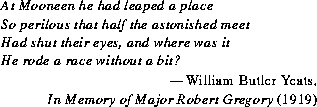# 21.3 The Class `java.util.Date`

The class `Date` provides a system-independent abstraction of dates and times, to a millisecond precision. Dates may be constructed from a year, month, date (day of month), hour, minute, and second; those six components and the day of the week, may be extracted; and dates may be compared and converted to a readable string.

```public class `Date` {
public `Date`();
public `Date`(long time);
public `Date`(int year, int month, int date);
public `Date`(int year, int month, int date,
int hours, int minutes);
public `Date`(int year, int month, int date,
int hours, int minutes, int seconds);
public `Date`(String s) throws IllegalArgumentException;
public String `toString`();
public boolean `equals`(Object obj);
public int `hashCode`();
public int `getYear`();
public void `setYear`(int year);
public int `getMonth`();
public void `setMonth`(int month);
public int `getDate`();
public void `setDate`(int date);
public int `getDay`();
public int `getHours`();
public void `setHours`(int hours);
public int `getMinutes`();
public void `setMinutes`(int minutes);
public int `getSeconds`();
public void `setSeconds`(int seconds);
public long `getTime`();
public void `setTime`(long time);
public boolean `before`(Date when);
public boolean `after`(Date when);
public String `toLocaleString`();
public String `toGMTString`();
public int `getTimezoneOffset`();
public static long `UTC`(int year, int month, int date,
int hours, int minutes, int seconds);
public static long `parse`(String s)
throws IllegalArgumentException;
}
```
Examples:

• To print today's date: System.out.println("today = " + new Date());
• To find out the day of the week for some particular date, for example, January 16, 1963: new Date(63, 0, 16).getDay()
While the `Date` class is intended to reflect UTC (Coordinated Universal Time), it may not do so exactly, depending on the host environment of the Java system. Nearly all modern operating systems assume that 1 day =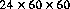= 86400 seconds in all cases. In UTC, however, about once every year or two there is an extra second, called a "leap second." The leap second is always added as the last second of the day, and nearly always on December 31 or June 30. For example, the last minute of the year 1995 was 61 seconds long, thanks to an added leap second.

Most computer clocks are currently not accurate enough to be able to reflect the leap-second distinction. Some computer standards are defined in terms of GMT (Greenwich Mean Time), which is equivalent to UT (Universal Time). GMT is the "civil" name for the standard; UT is the "scientific" name for the same standard. The distinction between UTC and UT is that UTC is based on an atomic clock and UT is based on astronomical observations, which for all practical purposes is an invisibly fine hair to split. Because the earth's rotation is not uniform-it slows down and speeds up in complicated ways-UT does not always flow uniformly. Leap seconds are introduced as needed into UTC so as to keep UTC within 0.9 seconds of UT1, which is a version of UT with certain corrections applied. There are other time and date systems as well; for example, the time scale used by GPS (the satellite-based Global Positioning System) is synchronized to UTC but is not adjusted for leap seconds. An interesting source of further information is the U. S. Naval Observatory, particularly the Directorate of Time at:

```http://tycho.usno.navy.mil
```
and their definitions of "Systems of Time" at:

```http://tycho.usno.navy.mil/systime.html
```
In all methods of class `Date` that accept or return year, month, day of month, hours, minutes, and seconds values, the following representations are used:

• A year y is represented by the integer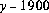.
• A month is represented by an integer form 0 to 11; 0 is January, 1 is February, and so on; thus 11 is December.
• A date (day of month) is represented by an integer from 1 to 31 in the usual manner.
• An hour is represented by an integer from 0 to 23. Thus the hour from midnight to 1 AM is hour 0, and the hour from noon to 1 PM is hour 12.
• A minute is represented by an integer from 0 to 59 in the usual manner.
• A second is represented by an integer from 0 to 61. The values 60 and 61 will occur only for leap seconds, and even then only in Java implementations that actually track leap seconds correctly. Because of the manner in which leap seconds are currently introduced, it is extremely unlikely that two leap seconds will occur in the same minute, but this specification follows the date and time conventions for ISO C.
In all cases, arguments given to methods for these purposes need not fall within the indicated ranges; for example, a date may be specified as January 32 and will be interpreted as meaning February 1.

21.3.1 `public Date()`

This constructor initializes a newly created `Date` object so that it represents the instant of time that it was created, measured to the nearest millisecond.

21.3.2 `public Date(long time)`

This constructor initializes a newly created `Date` object so that it represents the instant of time that is `time` milliseconds after the standard base time known as "the epoch," namely 00:00:00 GMT on January 1, 1970. See also the method `currentTimeMillis` (§20.18.6) of class `System`.

21.3.3 `public Date(int year, int month, int date)`

This constructor initializes a newly created `Date` object so that it represents midnight at the beginning of the day specified by the `year`, `month`, and `date` arguments, in the local time zone. Thus, it has the same effect as the constructor call (§21.3.5):

````Date(year, month, date, 0, 0, 0)`
```

21.3.4 `public Date(int year, int month, int date, int hours, int minutes)`

This constructor initializes a newly created `Date` object so that it represents the instant at the start of the minute specified by the `year`, `month`, `date`, `hours`, and `minutes` arguments, in the local time zone. Thus, it has the same effect as the constructor call (§21.3.5):

``````Date(year, month, date, hours, minutes, 0)
``````

21.3.5 `public Date(int year, int month, int date, int hours, int minutes, int seconds)`

This constructor initializes a newly created `Date` object so that it represents the instant at the start of the second specified by the `year`, `month`, `date`, `hours`, `minutes`, and `seconds` arguments, in the local time zone.

21.3.6 `public Date(String s)throws IllegalArgumentException`

This constructor initializes a newly created `Date` object so that it represents the date and time indicated by the string s, which is interpreted as if by the `parse` method (§21.3.31).

21.3.7 `public String toString()`

This `Date` object is converted to a `String` of the form:

```"dow mon dd hh:mm:ss zzz yyyy"
```
where:

• `dow` is the day of the week (`Sun`, `Mon`, `Tue`, `Wed`, `Thu`, `Fri`, `Sat`).
• `mon` is the month (`Jan`, `Feb`, `Mar`, `Apr`, `May`, `Jun`, `Jul`, `Aug`, `Sep`, `Oct`, `Nov`, `Dec`).
• `dd` is the day of the month (`01` through `31`), as two decimal digits.
• `hh` is the hour of the day (`00` through `23`), as two decimal digits.
• `mm` is the minute within the hour (`00` through `59`), as two decimal digits.
• `ss` is the second within the minute (`00` through `61`), as two decimal digits.
• `zzz` is the time zone (and may reflect daylight saving time). Standard time zone abbreviations include those recognized by the method `parse` (§21.3.31). If time zone information is not available, then `zzz` is empty-that is, it consists of no characters at all.
• `yyyy` is the year, as four decimal digits.
See also methods `toLocaleString` (§21.3.27) and `toGMTString` (§21.3.28).

Overrides the `toString` method of `Object` (§20.1.2).

21.3.8 `public boolean equals(Object obj)`

The result is `true` if and only if the argument is not `null` and is a `Date` object that represents the same point in time, to the millisecond, as this `Date` object. Thus two `Date` objects are equal if and only if the `getTime` method (§21.3.23) returns the same `long` value from both.

Overrides the `equals` method of `Object` (§20.1.3).

21.3.9 `public int hashCode()`

The result is the exclusive OR of the two halves of the primitive `long` value returned by the `getTime` method (§21.3.23). That is, the hash code is the value of the expression:

```(int)(this.getTime()^(this.getTime()>>>32))
```
Overrides the `hashCode` method of `Object` (§20.1.4).

21.3.10 `public int getYear()`

The returned value is the result of subtracting 1900 from the year that contains or begins with the instant in time represented by this `Date` object, as interpreted in the local time zone.

21.3.11 `public void setYear(int year)`

This `Date` object is modified so that it represents a point in time within the specified year, with the month, date, hour, minute, and second the same as before, as interpreted in the local time zone. (Of course, if the date was February 29, for example, and the year is set to a non-leap year, then the new date will be treated as if it were on March 1.)

21.3.12 `public int getMonth()`

The returned value is a number (`0` through `11`) representing the month that contains or begins with the instant in time represented by this `Date` object, as interpreted in the local time zone.

21.3.13 `public void setMonth(int month)`

This `Date` object is modified so that it represents a point in time within the specified month, with the year, date, hour, minute, and second the same as before, as interpreted in the local time zone. If the date was October 31, for example, and the month is set to June, then the new date will be treated as if it were on July 1, because June has only 30 days.

21.3.14 `public int getDate()`

The returned value is a number (`1` through `31`) representing day of the month that contains or begins with the instant in time represented by this `Date` object, as interpreted in the local time zone.

21.3.15 `public void setDate(int date)`

This `Date` object is modified so that it represents a point in time within the specified day of the month, with the year, month, hour, minute, and second the same as before, as interpreted in the local time zone.If the date was April 30, for example, and the date is set to 31, then it will be treated as if it were on May 1, because April has only 30 days.

21.3.16 `public int getDay()`

The returned value (`0` = Sunday, `1` = Monday, `2` = Tuesday, `3` = Wednesday, `4` = Thursday, `5` = Friday, `6` = Saturday) represents the day of the week that contains or begins with the instant in time represented by this `Date` object, as interpreted in the local time zone.

21.3.17 `public int getHours()`

The returned value is a number (`0` through `23`) representing the hour within the day that contains or begins with the instant in time represented by this `Date` object, as interpreted in the local time zone.

21.3.18 `public void setHours(int hours)`

This `Date` object is modified so that it represents a point in time within the specified hour of the day, with the year, month, date, minute, and second the same as before, as interpreted in the local time zone.

21.3.19 `public int getMinutes()`

The returned value is a number (`0` through `59`) representing the minute within the hour that contains or begins with the instant in time represented by this `Date` object, as interpreted in the local time zone.

21.3.20 `public void setMinutes(int minutes)`

This `Date` object is modified so that it represents a point in time within the specified minute of the hour, with the year, month, date, hour, and second the same as before, as interpreted in the local time zone.

21.3.21 `public int getSeconds()`

The returned value is a number (`0` through `61`) representing the second within the minute that contains or begins with the instant in time represented by this `Date` object, as interpreted in the local time zone.

21.3.22 `public void setSeconds(int seconds)`

This `Date` object is modified so that it represents a point in time within the specified second of the minute, with the year, month, date, hour, and minute the same as before, as interpreted in the local time zone.

21.3.23 `public long getTime()`

This method returns the time represented by this `Date` object, represented as the distance, measured in milliseconds, of that time from the epoch (00:00:00 GMT on January 1, 1970).

21.3.24 `public void setTime(long time)`

This `Date` object is modified so that it represents a point in time that is `time` milliseconds after the epoch (00:00:00 GMT on January 1, 1970).

21.3.25 `public boolean before(Date when)`

The result is `true` if and only if the instant represented by this `Date` object is strictly earlier than the instant represented by `when`.

21.3.26 `public boolean after(Date when)`

The result is `true` if and only if the instant represented by this `Date` object is strictly later than the instant represented by `when`.

21.3.27 `public String toLocaleString()`

This `Date` object is converted to a `String` of an implementation-dependent form. The general intent is that the form should be familiar to the user of the Java application, wherever it may happen to be running. The intent is comparable to that of the `%c` format supported by the `strftime` function of ISO C.

See also methods `toString` (§21.3.7) and `toGMTString` (§21.3.28).

21.3.28 `public String toGMTString()`

This `Date` object is converted to a `String` of length 23 or 24 of the form:

```"d mon yyyy hh:mm:ss GMT"
```
where:

• d is the day of the month (`1` through `31`), as one or two decimal digits.
• mon is the month (`Jan`, `Feb`, `Mar`, `Apr`, `May`, `Jun`, `Jul`, `Aug`, `Sep`, `Oct`, `Nov`, `Dec`).
• yyyy is the year, as four decimal digits.
• hh is the hour of the day (`00` through `23`), as two decimal digits.
• mm is the minute within the hour (`00` through `59`), as two decimal digits.
• ss is the second within the minute (`00` through `61`), as two decimal digits.
• GMT is exactly the ASCII letters "`GMT`" to indicate Greenwich Mean Time.
The result does not depend on the local time zone.

See also methods `toString` (§21.3.7) and `toLocaleString` (§21.3.27).

21.3.29 `public int getTimezoneOffset()`

This method returns the offset, measured in minutes, for the local time zone relative to UTC that is appropriate for the time represented by this `Date` object.

For example, in Massachusetts, five time zones west of Greenwich:

```new Date(96, 1, 14).getTimezoneOffset() returns 300
```
because on February 14, 1996, standard time (Eastern Standard Time) is in use, which is offset five hours from UTC; but:

```new Date(96, 5, 1).getTimezoneOffset() returns 240
```
because on May 1, 1996, daylight saving time (Eastern Daylight Time) is in use, which is offset only four hours from UTC.

This method produces the same result as if it computed:

```
(this.getTime() - UTC(this.getYear(),
this.getMonth(),
this.getDate(),
this.getHours(),
this.getMinutes(),
this.getSeconds())) / (60 * 1000)
```

21.3.30 `public static long UTC(int year, int month, int date, int hours, int minutes, int seconds)`

The arguments are interpreted as a year, month, day of the month, hour of the day, minute within the hour, and second within the minute, exactly as for the `Date` constructor of six arguments (§21.3.5), except that the arguments are interpreted relative to UTC rather than to the local time zone. The time indicated is returned represented as the distance, measured in milliseconds, of that time from the epoch (00:00:00 GMT on January 1, 1970).

21.3.31 `public static long parse(String s)throws IllegalArgumentException`

An attempt is made to interpret the string `s` as a representation of a date and time. If the attempt is successful, the time indicated is returned represented as the distance, measured in milliseconds, of that time from the epoch (00:00:00 GMT on January 1, 1970). If the attempt fails, an `IllegalArgumentException` is thrown.

The string `s` is processed from left to right, looking for data of interest.

Any material in `s` that is within the ASCII parenthesis characters `(` and `)` is ignored. Parentheses may be nested. Otherwise, the only characters permitted within `s` are these ASCII characters:

```
abcdefghijklmnopqrstuvwxyz
ABCDEFGHIJKLMNOPQRSTUVWXYZ
0123456789,+-:/
```
and whitespace characters (§20.5.19).

A consecutive sequence of decimal digits is treated as a decimal number:

• If a number is preceded by `+` or `-` and a year has already been recognized, then the number is a time-zone offset. If the number is less than 24, it is an offset measured in hours. Otherwise, it is regarded as an offset in minutes, expressed in 24-hour time format without punctuation. A preceding `+` means an eastward offset and a preceding `-` means a westward offset. Time zone offsets are always relative to UTC (Greenwich). Thus, for example, `-5` occurring in the string would mean "five hours west of Greenwich" and `+0430` would mean "four hours and thirty minutes east of Greenwich." It is permitted for the string to specify `GMT`, `UT`, or `UTC` redundantly-for example, `GMT-5` or `utc+0430`.
• If a number is greater than 70, it is regarded as a year number. It must be followed by a space, comma, slash, or end of string. If it is greater than 1900, then 1900 is subtracted from it.
• If the number is followed by a colon, it is regarded as an hour, unless an hour has already been recognized, in which case it is regarded as a minute.
• If the number is followed by a slash, it is regarded as a month (it is decreased by `1` to produce a number in the range `0` to `11`), unless a month has already been recognized, in which case it is regarded as a day of the month.
• If the number is followed by whitespace, a comma, a hyphen, or end of string, then if an hour has been recognized but not a minute, it is regarded as a minute; otherwise, if a minute has been recognized but not a second, it is regarded as a second; otherwise, it is regarded as a day of the month.
A consecutive sequence of letters is regarded as a word and treated as follows:

• A word that matches `AM`, ignoring case, is ignored (but the parse fails if an hour has not been recognized or is less than `1` or greater than `12`).
• A word that matches `PM`, ignoring case, adds `12` to the hour (but the parse fails if an hour has not been recognized or is less than `1` or greater than `12`).
• Any word that matches any prefix of `SUNDAY`, `MONDAY`, `TUESDAY`, `WEDNESDAY`, `THURSDAY`, `FRIDAY`, or `SATURDAY`, ignoring case, is ignored. For example, `sat`, `Friday`, `TUE`, and `Thurs` are ignored.
• Otherwise, any word that matches any prefix of `JANUARY`, `FEBRUARY`, `MARCH`, `APRIL`, `MAY`, `JUNE`, `JULY`, `AUGUST`, `SEPTEMBER`, `OCTOBER`, `NOVEMBER`, or `DECEMBER`, ignoring case, and considering them in the order given here, is recognized as specifying a month and is converted to a number (`0` to `11`). For example, `aug`, `Sept`, `april`, and `NOV` are recognized as months. So is `Ma`, which is recognized as `MARCH`, not `MAY`.
• Any word that matches `GMT`, `UT`, or `UTC`, ignoring case, is treated as referring to UTC.
• Any word that matches `EST`, `CST`, `MST`, or `PST`, ignoring case, is recognized as referring to the time zone in North America that is five, six, seven, or eight hours west of Greenwich, respectively. Any word that matches `EDT`, `CDT`, `MDT`, or `PDT`, ignoring case, is recognized as referring to the same time zone, respectively, during daylight saving time. (In the future, this method may be upgraded to recognize other time zone designations.)
Once the entire string `s` has been scanned, it is converted to a time result in one of two ways. If a time zone or time-zone offset has been recognized, then the year, month, day of month, hour, minute, and second are interpreted in UTC (§21.3.30) and then the time-zone offset is applied. Otherwise, the year, month, day of month, hour, minute, and second are interpreted in the local time zone.

# 21.4 The Class `java.util.Dictionary`

A `Dictionary` is an object that associates elements with keys. Every key and every element is an object. In any one `Dictionary`, every key is associated at most one element. Given a `Dictionary` and a key, the associated element can be looked up.

```public abstract class `Dictionary` {
abstract public int `size`();
abstract public boolean `isEmpty`();
abstract public Object `get`(Object key)
throws NullPointerException;
abstract public Object `put`(Object key, Object element)
throws NullPointerException;
abstract public Object `remove`(Object key)
throws NullPointerException;
abstract public Enumeration `keys`();
abstract public Enumeration `elements`();
}
```
As a rule, the `equals` method (§20.1.3) should be used by implementations of the class `Dictionary` to decide whether two keys are the same.

21.4.1 `abstract public int size()`

The general contract for the `size` method is that it returns the number of entries (distinct keys) in this dictionary.

21.4.2 `abstract public boolean isEmpty()`

The general contract for the `isEmpty` method is that the result is `true` if and only if this dictionary contains no entries.

21.4.3 `abstract public Object get(Object key)throws NullPointerException`

The general contract for the `isEmpty` method is that if this dictionary contains an entry for the specified `key`, the associated element is returned; otherwise, `null` is returned.

If the `key` is `null`, a `NullPointerException` is thrown.

21.4.4 `abstract public Object put(Object key, Object element)throws NullPointerException`

The general contract for the `put` method is that it adds an entry to this dictionary.

If this dictionary already contains an entry for the specified `key`, the element already in this dictionary for that `key` is returned, after modifying the entry to contain the new `element`.

If this dictionary does not already have an entry for the specified `key`, an entry is created for the specified `key` and `element`, and `null` is returned.

If the `key` or the `element` is `null`, a `NullPointerException` is thrown.

21.4.5 `abstract public Object remove(Object key)throws NullPointerException`

The general contract for the `remove` method is that it removes an entry from this dictionary.

If this dictionary contains an entry for the specified `key`, the element in this dictionary for that `key` is returned, after removing the entry from this dictionary.

If this dictionary does not already have an entry for the specified `key`, `null` is returned.

If the `key` is `null`, a `NullPointerException` is thrown.

21.4.6 `abstract public Enumeration keys()`

The general contract for the `keys` method is that an `Enumeration` (§21.1) is returned that will generate all the keys for which this dictionary contains entries.

21.4.7 `abstract public Enumeration elements()`

The general contract for the `elements` method is that an `Enumeration` (§21.1) is returned that will generate all the elements contained in entries in this dictionary.

# 21.5 The Class `java.util.Hashtable`

The class `Hashtable` implements the abstract class `Dictionary` (§21.4), with some additional functionality.

```public class `Hashtable` extends Dictionary implements Cloneable {
public `Hashtable`(int initialCapacity, float loadFactor);
public `Hashtable`(int initialCapacity);
public `Hashtable`();
public String `toString`();
public Object `clone`();
public int `size`();
public boolean `isEmpty`();
public Object `get`(Object key)
throws NullPointerException;
public Object `put`(Object key, Object value)
throws NullPointerException;
public Object `remove`(Object key)
throws NullPointerException;
public Enumeration `keys`();
public Enumeration `elements`();
public boolean `contains`(Object value);
public boolean `containsKey`(Object key);
protected void `rehash`();
public void `clear`();
}
```
A `Hashtable` has two parameters that affect its efficiency: its capacity and its load factor. The load factor should be between `0.0` and `1.0`. When the number of entries in the hashtable exceeds the product of the load factor and the current capacity, the capacity is increased, using the `rehash` method. Larger load factors use memory more efficiently at the expense of larger expected time per lookup. If many entries are to be made in a `Hashtable`, creating it with a sufficiently large capacity may allow the entries to be inserted more efficiently than letting it perform automatic rehashing as needed to grow the table.

21.5.1 `public Hashtable(int initialCapacity, float loadFactor)`

This constructor initializes a newly created `Hashtable` object so that its capacity is `initialCapacity` and its load factor is `loadFactor`. Initially, there are no entries in the table.

21.5.2 `public Hashtable(int initialCapacity)`

This constructor initializes a newly created `Hashtable` object so that its capacity is `initialCapacity` and its load factor is `0.75`. Initially, there are no entries in the table.

21.5.3 `public Hashtable()`

This constructor initializes a newly created `Hashtable` object so that its load factor is `0.75`. Initially, there are no entries in the table.

21.5.4 `public String toString()`

This `Hashtable` is represented in string form as a set of entries, enclosed in braces and separated by the ASCII characters "`, `" (comma and space). Each entry is rendered as the key, an equals sign `=`, and the associated element, where the `toString` method is used to convert the key and element to strings.

Overrides the `toString` method of `Object` (§21.2.3).

21.5.5 `public Object clone()`

A copy of this `Hashtable` is constructed and returned. All the structure of the hashtable itself is copied, but the keys and elements are not cloned.

Overrides the `clone` method of `Object` (§21.2.6).

21.5.6 `public int size()`

Implements the `size` method of `Dictionary` (§21.4.1).

21.5.7 `public boolean isEmpty()`

Implements the `isEmpty` method of `Dictionary` (§21.4.2).

21.5.8 `public Object get(Object key)`

Implements the `get` method of `Dictionary` (§21.4.3).

21.5.9 `public Object put(Object key, Object value)`

Implements the `put` method of `Dictionary` (§21.4.4).

21.5.10 `public Object remove(Object key)`

Implements the `remove` method of `Dictionary` (§21.4.5).

21.5.11 `public Enumeration keys()`

Implements the `keys` method of `Dictionary` (§21.4.6).

21.5.12 `public Enumeration elements()`

Implements the `elements` method of `Dictionary` (§21.4.7).

21.5.13 `public boolean contains(Object value)`

The result is `true` if and only if this `Hashtable` contains at least one entry for which the element is equal to `value`, as determined by the `equals` method (§20.1.3).

21.5.14 `public boolean containsKey(Object key)`

The result is `true` if and only if this `Hashtable` contains an entry for which the key is equal to `key`, as determined by the `equals` method (§20.1.3). In other words, this method produces the same result as the expression:

```get(key) != null
```

21.5.15 `protected void rehash()`

This `Hashtable` is increased in capacity and reorganized internally, in order to accommodate and access its entries more efficiently.

21.5.16 `public void clear()`

The `clear` method removes all entries from this `Hashtable`.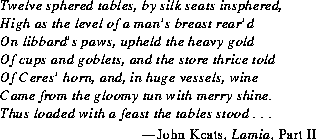# 21.6 The Class `java.util.Properties`

A `Properties` table is a kind of `Hashtable` with two functionality extensions and with the restriction that keys and elements must be strings. First, there are methods for reading entries into the table from an input stream and writing all the entries in the table to an output stream. Second, a `Properties` table may refer to another `Properties` table that provides default values. The `getProperty` method is much like the `get` method (§21.4.3), but if an entry is not found in this table, then the defaults table is searched (and that defaults table may itself refer to another defaults table, and so on, recursively).

```public class `Properties` extends Hashtable {
protected Properties `defaults`;
public `Properties`();
public `Properties`(Properties defaults);
public String `getProperty`(String key);
public String `getProperty`(String key, String defaultValue);
public Enumeration `propertyNames`();
public void `load`(InputStream in) throws IOException;
public void `save`(OutputStream out, String header);
public void `list`(PrintStream out);
}
```

21.6.1 `protected Properties defaults;`

If the `defaults` field is not `null`, it is another `Properties` table that provides default values for this `Properties` table.

21.6.2 `public Properties()`

This constructor initializes a newly created `Properties` table so that it has no defaults table. Initially, there are no entries in the newly created table.

21.6.3 `public Properties(Properties defaults)`

This constructor initializes a newly created `Properties` table so its defaults table is `defaults`. The argument `defaults` may be `null`, in which case the newly created `Properties` table will not have a defaults table. Initially, there are no entries in the newly created table.

21.6.4 `public String getProperty(String key)`

If there is an entry in this `Properties` table with `key` as its key, the associated element is returned. Otherwise, if this `Properties` table has a defaults table, then whatever its `getProperty` method returns is returned. Otherwise, `null` is returned.

21.6.5 `public String getProperty(String key, String defaultValue)`

If there is an entry in this `Properties` table with `key` as its key, the associated element is returned. Otherwise, if this `Properties` table has a defaults table, then whatever its `getProperty` method returns is returned. Otherwise, `defaultValue` is returned.

21.6.6 `public Enumeration propertyNames()`

An `Enumeration` (§21.1) is returned that will generate all the keys for which this `Properties` table could supply an associated element. If this `Properties` table has a defaults table (§21.6.1), then keys for which the defaults table has entries are also supplied by the `Enumeration`, and so on, recursively; but no key is supplied by the `Enumeration` more than once.

21.6.7 `public void load(InputStream in) throws IOException`

Properties (key and element pairs) are read from the input stream:

```Runtime.getRuntime().getLocalizedInputStream(in)
```
and added to this `Properties` table. See the `getLocalizedInputStream` method of `Runtime` (§20.16.15).

Every property occupies one line of the input stream. Each line is terminated by a line terminator (`\n` or `\r` or `\r\n`). Lines from the input stream are processed until end of file is reached on the input stream.

A line that contains only whitespace (§20.5.19) or whose first non-whitespace character is an ASCII `#` or `!` is ignored (thus, `#` or `!` indicate comment lines).

Every line other than a blank line or a comment line describes one property to be added to the table (except that if a line ends with \, then the following line is treated as a continuation line, as described below). The key consists of all the characters in the line starting with the first non-whitespace character and up to, but not including, the first ASCII `=`, `:`, or whitespace character. Any whitespace after the key is skipped; if the first non-whitespace character after the key is `=` or `:`, then it is ignored and any whitespace characters after it are also skipped. All remaining characters on the line become part of the associated element string. Within the element string (but not the key), the ASCII escape sequences `\t`, `\n`, `\r`, `\\`, `\"`, `\'`, `\ ` (a backslash and a space), and `\u`xxxx are recognized and converted to single characters. Moreover, if the last character on the line is `\`, then the next line is treated as a continuation of the current line; the `\` and line terminator are simply discarded, and any leading whitespace characters on the continuation line are also discarded and are not part of the element string.

As an example, each of the following four lines specifies the key `"Truth"` and the associated element value `"Beauty"`:

```
Truth Beauty
Truth = Beauty
Truth:Beauty
Truth			:Beauty
```
As another example, the following three lines specify a single property:

```
fruits				apple, banana, pear, \
cantaloupe, watermelon, \
kiwi, mango
```
The key is `"fruit"` and the associated element is:

```"apple, banana, pear, cantaloupe, watermelon, kiwi, mango"
```
Note that a space appears before each `\` so that a space will appear after each comma in the final result; the `\`, line terminator, and leading whitespace on the continuation line are merely discarded and are not replaced by one or more other characters.

As a third example, the line:

```cheeses
```
specifies that the key is `"cheeses"` and the associated element is the empty string.

21.6.8 `public void save(OutputStream out, String header)`

All the properties (key and element pairs) in this `Properties` table are written to the output stream:

```Runtime.getRuntime().getLocalizedOutputStream(out)
```
in a format suitable for loading into a `Properties` table using the `load` method (§21.6.7). See the `getLocalizedOutputStream` method of `Runtime` (§20.16.16).

Properties from the defaults table of this `Properties` table (if any) are not written out by this method.

If the header argument is not null, then an ASCII `#` character, the header string, and a newline are first written to the output stream. Thus, the `header` can serve as an identifying comment.

Next, a comment line is always written, consisting of an ASCII `#` character, the current date and time (as if produced by the `toString` method of `Date` (§21.3.7) for the current time), and a newline.

Then every entry in this `Properties` table is written out, one per line. For each entry the key string is written, then an ASCII `=`, then the associated element string. Each character of the element string is examined to see whether it should be rendered as an escape sequence. The ASCII characters `\`, tab, newline, and carriage return are written as `\\`, `\t`, `\n`, and `\r`, respectively. Characters less than `\u0020` and characters greater than `\u007E` (if necessary, depending on the needs of the localized output stream) are written as `\u`xxxx for the appropriate hexadecimal value xxxx. Leading space characters, but not embedded or trailing space characters, are written with a preceding `\`.

21.6.9 `public void list(PrintStream out)`

Properties (key and element pairs) in this `Properties` table are written to the output stream `out` in a possibly abbreviated form that may be more convenient for use in debugging than the output of the `save` method. No header is written, and element values longer than 40 character are truncated to the first 37 characters, to which the characters "`...`" are appended. Thus, if the names of the keys are not too long, there is a fighting chance that each property will fit into the space of one line of a physical output device.

# 21.7 The Class `java.util.Observable`

Each instance of class `Observable` maintains a set of "observers" that are notified whenever the `Observable` object changes in some significant way. An observer may be any object that implements interface `Observer` (§21.8).

Note that this notification mechanism is has nothing to do with threads (§20.20) and is completely separate from the `wait` and `notify` mechanism of class `Object` (§20.1).

```public class `Observable` {
public void `addObserver`(Observer o);
public void `deleteObserver`(Observer o);
public void `deleteObservers`();
public int `countObservers`();
public void `notifyObservers`();
public void `notifyObservers`(Object arg);
protected void `setChanged`();
protected void `clearChanged`();
public boolean `hasChanged`();
}
```
When an observable object is newly created, its set of observers is empty.

Two observers are considered the same if and only if the `equals` method (§20.1.3) returns `true` for them.

21.7.1 `public void addObserver(Observer o)`

The observer `o` is added to this `Observable` object's set of observers, provided that it is not the same as some observer already in the set.

21.7.2 `public void deleteObserver(Observer o)`

The observer `o` is removed from this `Observable` object's set of observers.

21.7.3 `public void deleteObservers()`

All observers are removed from this `Observable` object's set of observers.

21.7.4 `public int countObservers()`

The number of observers in this `Observable` object's set of observers is returned.

21.7.5 `public void notifyObservers()`

If this `Observable` object has been marked as changed, this method causes all observers to be notified with `null` as the second argument; in other words, this method is equivalent to:

```notifyObservers(null)
```

21.7.6 `public void notifyObservers(Object arg)`

If this `Observable` object has been marked as changed (§21.7.9), this method causes all observers to be notified with `arg` as the second argument. An observer is notified by calling its `update` method (§21.8.1) on two arguments: this `Observable` object and `arg`. The mark on this object is then cleared (§21.7.8).

21.7.7 `protected void setChanged()`

This `Observable` object is marked as having been changed; the `hasChanged` method will now return `true`.

21.7.8 `protected void clearChanged()`

This `Observable` object is marked as not having been changed; the `hasChanged` method will now return `false`.

21.7.9 `public boolean hasChanged()`

The result is true if and only if the `setChanged` method has been called for this `Observable` object more recently than either the `clearChanged` method or the `notifyObservers` method.

# 21.8 The Interface `java.util.Observer`

A class should implement the `Observer` interface if it is to be notified whenever an `Observable` object has been changed. See the `Observable` class (§21.7) for a discussion of how `Observer` objects are notified.

```public interface `Observer` {
public void `update`(Observable o, Object arg);
}
```

21.8.1 `public void update(Observable o, Object arg)`

When an `Observable` object has been changed and its `notifyObservers` method (§21.7.6) is called, every `Observer` object in its set of `observers` is notified by invoking its `update` method, passing it two arguments: the `Observable` object and another argument specified by the call to the `notifyObservers` method.

# 21.9 The Class `java.util.Random`

Each instance of class `Random` serves as a separate, independent pseudorandom generator of primitive values.

```public class `Random` {
protected long `seed`;
protected double `nextNextGaussian`;
protected boolean `haveNextNextGaussian` = false;
public `Random`();
public `Random`(long seed);
public void `setSeed`(long seed);
protected int `next`(int bits);
public int `nextInt`();
public long `nextLong`();
public float `nextFloat`();
public double `nextDouble`();
public double `nextGaussian`();
}
```
If two `Random` objects are created with the same seed and the same sequence of method calls is made for each, they will generate and return identical sequences of numbers in all Java implementations. In order to guarantee this property, particular algorithms are specified for the class `Random`. Java implementations must use all the algorithms shown here for the class `Random`, for the sake of absolute portability of Java code. However, subclasses of class `Random` are permitted use other algorithms, so long as they adhere to the general contracts for all the methods.

The algorithms implemented by class `Random` use three state variables, which are `protected`. They also use a `protected` utility method that on each invocation can supply up to up to 32 pseudorandomly generated bits.

21.9.1 `protected long seed;`

A variable used by method `next` (§21.9.7) to hold the state of the pseudorandom number generator.

21.9.2 `protected double nextNextGaussian;`

A variable used by method `nextGaussian` (§21.9.12) to hold a precomputed value to be delivered by that method the next time it is called.

21.9.3 `protected boolean haveNextNextGaussian = false;`

A variable used by method `nextGaussian` (§21.9.12) to keep track of whether it has precomputed and stashed away the next value to be delivered by that method.

21.9.4 `public Random()`

This constructor initializes a newly created `Random` number generator by using the current time of day (§20.18.6) as a seed.

```public Random() { this(System.currentTimeMillis()); }
```

21.9.5 `public Random(long seed)`

This constructor initializes a newly created `Random` number generator by using the argument `seed` as a seed.

```
public Random(long seed) { setSeed(seed); }
```

21.9.6 `public void setSeed(long seed)`

The general contract of `setSeed` is that it alters the state of this random number generator object so as to be in exactly the same state as if it had just been created with the argument `seed` as a seed.

The method `setSeed` is implemented by class `Random` as follows:

```
synchronized public void setSeed(long seed) {
this.seed = (seed ^ 0x5DEECE66DL) & ((1L << 48) - 1);
haveNextNextGaussian = false;
}
```
The implementation of `setSeed` by class `Random` happens to use only 48 bits of the given seed. In general, however, an overriding method may use all 64 bits of the long argument as a seed value.

[In certain early versions of Java, the `setSeed` method failed to reset the value of `haveNextNextGaussian` to `false`; this flaw could lead to failure to produce repeatable behavior.]

21.9.7 `protected int next(int bits)`

The general contract of `next` is that it returns an `int` value and if the argument bits is between `1` and `32` (inclusive), then that many low-order bits of the returned value will be (approximately) independently chosen bit values, each of which is (approximately) equally likely to be `0` or `1`.

The method `next` is implemented by class `Random` as follows:

```
synchronized protected int next(int bits) {
seed = (seed * 0x5DEECE66DL + 0xBL) & ((1L << 48) - 1);
return (int)(seed >>> (48 - bits));
}
```
This is a linear congruential pseudorandom number generator, as defined by D. H. Lehmer and described by Donald E. Knuth in The Art of Computer Programming, Volume 2: Seminumerical Algorithms, section 3.2.1.

21.9.8 `public int nextInt()`

The general contract of `nextInt` is that one `int` value is pseudorandomly generated and returned. All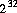possible `int` values are produced with (approximately) equal probability.

The method `setSeed` is implemented by class `Random` as follows:

```public int nextInt() {  return next(32); }
```

21.9.9 `public long nextLong()`

The general contract of `nextLong` is that one `long` value is pseudorandomly generated and returned. All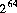possible `long` values are produced with (approximately) equal probability.

The method `setSeed` is implemented by class `Random` as follows:

```
public long nextLong() {
return ((long)next(32) << 32) + next(32);
}
```

21.9.10 `public float nextFloat()`

The general contract of `nextFloat` is that one `float` value, chosen (approximately) uniformly from the range `0.0f` (inclusive) to `1.0f` (exclusive), is pseudorandomly generated and returned. All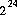possible `float` values of the form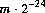, where m is a positive integer less than, are produced with (approximately) equal probability.

The method `setSeed` is implemented by class `Random` as follows:

```
public float nextFloat() {
return next(24) / ((float)(1 << 24));
}
```
The hedge "approximately" is used in the foregoing description only because the `next` method is only approximately an unbiased source of independently chosen bits. If it were a perfect source or randomly chosen bits, then the algorithm shown would choose `float` values from the stated range with perfect uniformity.

[In early versions of Java, the result was incorrectly calculated as:

```	return next(30) / ((float)(1 << 30));
```
This might seem to be equivalent, if not better, but in fact it introduced a slight nonuniformity because of the bias in the rounding of floating-point numbers: it was slightly more likely that the low-order bit of the significand would be `0` than that it would be `1`.]

21.9.11 `public double nextDouble()`

The general contract of `nextDouble` is that one `double` value, chosen (approximately) uniformly from the range `0.0d` (inclusive) to `1.0d` (exclusive), is pseudorandomly generated and returned. All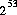possible `float` values of the form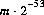, where m is a positive integer less than, are produced with (approximately) equal probability.

The method `setSeed` is implemented by class `Random` as follows:

```
public double nextDouble() {
return (((long)next(26) << 27) + next(27))
/ (double)(1L << 53);
}
```
The hedge "approximately" is used in the foregoing description only because the `next` method is only approximately an unbiased source of independently chosen bits. If it were a perfect source or randomly chosen bits, then the algorithm shown would choose `double` values from the stated range with perfect uniformity.

[In early versions of Java, the result was incorrectly calculated as:

```
return (((long)next(27) << 27) + next(27))
/ (double)(1L << 54);
```
This might seem to be equivalent, if not better, but in fact it introduced a large nonuniformity because of the bias in the rounding of floating-point numbers: it was three times as likely that the low-order bit of the significand would be `0` than that it would be `1`! This nonuniformity probably doesn't matter much in practice, but we strive for perfection.]

21.9.12 `public double nextGaussian()`

The general contract of `nextGaussian` is that one `double` value, chosen from (approximately) the usual normal distribution with mean `0.0` and standard deviation `1.0`, is pseudorandomly generated and returned.

The method `setSeed` is implemented by class `Random` as follows:

```
synchronized public double nextGaussian() {
if (haveNextNextGaussian) {
haveNextNextGaussian = false;
return nextNextGaussian;
} else {
double v1, v2, s;
do {
v1 = 2 * nextDouble() - 1; 	// between -1.0 and 1.0
v2 = 2 * nextDouble() - 1; 	// between -1.0 and 1.0
s = v1 * v1 + v2 * v2;
} while (s >= 1);
double norm = Math.sqrt(-2 * Math.log(s)/s);
nextNextGaussian = v2 * norm;
haveNextNextGaussian = true;
return v1 * norm;
}
}
```
This uses the polar method of G. E. P. Box, M. E. Muller, and G. Marsaglia, as described by Donald E. Knuth in The Art of Computer Programming, Volume 2: Seminumerical Algorithms, section 3.4.1, subsection C, algorithm P. Note that it generates two independent values at the cost of only one call to `Math.log` and one call to `Math.sqrt`.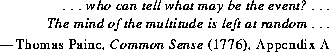# 21.10 The Class `java.util.StringTokenizer`

The `StringTokenizer` class provides a way to break a `String` into tokens. The tokenizing method used by this class is much simpler than the one used by the class `java.io.StreamTokenizer`. For example, a `StringTokenizer` does not distinguish among identifiers, numbers, and quoted strings; moreover, it does not recognize and skip comments.

A `StringTokenizer` can serve as an `Enumeration` (§21.1).

```public class `StringTokenizer` implements Enumeration {
public `StringTokenizer`(String str, String delim,
boolean returnTokens);
public `StringTokenizer`(String str, String delim);
public `StringTokenizer`(String str);
public boolean `hasMoreTokens`();
public String `nextToken`();
public String `nextToken`(String delim);
public boolean `hasMoreElements`();
public Object `nextElement`();
public int `countTokens`();
}
```
A `StringTokenizer` simply divides characters into classes: delimiters and other characters. The tokenizer behaves in one of two ways, depending on whether it was created with `returnTokens` having the value `true` or `false`.

If `returnTokens` is `false`, delimiter characters merely serve to separate tokens of interest. A token is thus a maximal sequence of consecutive characters that are not delimiters.

If `returnTokens` is `true`, delimiter characters are themselves considered to be tokens of interest. A token is thus either one delimiter character or a maximal sequence of consecutive characters that are not delimiters.

A `StringTokenizer` internally maintains a current position within the `String` to be tokenized. Some operations advance this current position past the characters processed.

A token is returned by taking a substring (§20.12.32) of the string that was used to create the `StringTokenizer`.

21.10.1 `public StringTokenizer(String str, String delim, boolean returnTokens)`

This constructor initializes a newly created `StringTokenizer` so that it will recognize tokens within the given string `str`. All characters in the string `delim` will be considered delimiters. The argument `returnTokens` specifies whether delimiter characters themselves are to be considered tokens.

21.10.2 `public StringTokenizer(String str, String delim)`

This constructor initializes a newly created `StringTokenizer` so that it will recognize tokens within the given string `str`. All characters in the string `delim` will be considered delimiters. Delimiter characters themselves will not be treated as tokens.

21.10.3 `public StringTokenizer(String str)`

This constructor initializes a newly created `StringTokenizer` so that it will recognize tokens within the given string `str`. All whitespace characters (§20.5.19) will be considered delimiters. Delimiter characters themselves will not be treated as tokens.

21.10.4 `public boolean hasMoreTokens()`

The result is `true` if and only if there is at least one token in the string after the current position. If this method returns `true`, then a subsequent call to `nextToken` with no argument will successfully return a token.

21.10.5 `public String nextToken()`

The next token in the string after the current position is returned. The current position is advanced beyond the recognized token.

21.10.6 `public String nextToken(String delim)`

First, the set of characters considered to be delimiters by this `StringTokenizer` is changed to be the characters in the string `delim`. Then the next token in the string after the current position is returned. The current position is advanced beyond the recognized token.

21.10.7 `public boolean hasMoreElements()`

This method has exactly the same behavior as `hasMoreTokens` (§21.10.4). It is provided so that a `StringTokenizer` can serve as an `Enumeration` (§21.1).

21.10.8 `public Object nextElement()`

This method has exactly the same behavior as `nextToken` (§21.10.5). It is provided so that a `StringTokenizer` can serve as an `Enumeration` (§21.1).

21.10.9 `public int countTokens()`

The result is the number of tokens in the string after the current position, using the current set of delimiter characters. The current position is not advanced.

# 21.11 The Class `java.util.Vector`

A `Vector`, like an array, contains items that can be accessed using an integer index. However, the size of a `Vector` can grow and shrink as needed to accommodate adding and removing items after the `Vector` has been created.

```public class `Vector` implements Cloneable {
protected Object[] `elementData`;
protected int `elementCount`;
protected int `capacityIncrement`;
public `Vector`(int initialCapacity, int capacityIncrement);
public `Vector`(int initialCapacity);
public `Vector`();
public final String `toString`();
public Object `clone`();
public final Object `elementAt`(int index)
throws `IndexOutOfBoundsException`;
public final void `setElementAt`(Object obj, int index)
throws `IndexOutOfBoundsException`;
public final Object `firstElement`()
throws NoSuchElementException;
public final Object `lastElement`()
throws NoSuchElementException;
public final void `addElement`(Object obj);
public final void `insertElementAt`(Object obj, int index)
throws `IndexOutOfBoundsException`;
public final boolean `removeElement`(Object obj);
public final void `removeElementAt`(int index)
throws `IndexOutOfBoundsException`;
public final void `removeAllElements`();
public final boolean `isEmpty`();
public final int `size`();
public final void `setSize`(int newSize);
public final int `capacity`();
public final void `ensureCapacity`(int minCapacity);
public final void `trimToSize`();
public final void `copyInto`(Object anArray[])
throws `IndexOutOfBoundsException`;
public final Enumeration `elements`();
public final boolean `contains`(Object elem);
public final int `indexOf`(Object elem);
public final int `indexOf`(Object elem, int index)
throws `IndexOutOfBoundsException`;
public final int `lastIndexOf`(Object elem);
public final int `lastIndexOf`(Object elem, int index)
throws `IndexOutOfBoundsException`;
}
```

21.11.1 `protected Object[] elementData;`

Internally, a `Vector` keeps its elements in an array that is at least large enough to contain all the elements.

21.11.2 `protected int elementCount;`

This field holds the number of items currently in this `Vector` object. Components `elementData` through `elementData[elementCount-1]` are the actual items.

21.11.3 `protected int capacityIncrement;`

When the method `ensureCapacity` (§21.11.22) must increase the size of the data array in the field `elementData` (by creating a new array), it increases the size by at least the amount in `capacityIncrement`; but if `capacityIncrement` is zero, then it at least doubles the size of the data array.

21.11.4 `public Vector(int initialCapacity, int capacityIncrement)`

This constructor initializes a newly created `Vector` so that its internal data array has size `initialCapacity` and its standard capacity increment is the value of `capacityIncrement`. Initially, the `Vector` contains no items.

21.11.5 `public Vector(int initialCapacity)`

This constructor initializes a newly created `Vector` so that its internal data array has size `initialCapacity` and its standard capacity increment is zero. Initially, the `Vector` contains no items.

21.11.6 `public Vector()`

This constructor initializes a newly created `Vector` so that its internal data array has size `10` and its standard capacity increment is zero. Initially the `Vector` contains no items.

21.11.7 `public final String toString()`

This `Vector` is represented in string form as a list of its items, enclosed in ASCII square brackets and separated by the ASCII characters "`, `" (comma and space). The `toString` method is used to convert the items to strings; a null reference is rendered as the string "`null`".

The example fragment:

```
Vector v = new Vector();
System.out.println(v.toString());

```
produces the output:

```[Canberra, Cancun, Canandaigua]
```
Overrides the `toString` method of `Object` (§20.1.2).

21.11.8 `public Object clone()`

A copy of this `Vector` is constructed and returned. The copy will contains a reference to a clone of the internal data array, not a reference to the original internal data array of this `Vector`.

Overrides the `clone` method of `Object` (§20.1.5).

21.11.9 `public final Object elementAt(int index)throws IndexOutOfBoundsException`

The item of this `Vector` with the specified `index` is returned.

If the `index` is negative or not less than the current size of this `Vector`, an `IndexOutOfBoundsException` is thrown.

21.11.10 `public final void setElementAt(Object obj, int index)throws IndexOutOfBoundsException`

The item of this `Vector` with the specified `index` is replaced with `obj`, so that `obj` is now the item at the specified `index` within this `Vector`.

If the `index` is negative or not less than the current size of this `Vector`, an `IndexOutOfBoundsException` is thrown.

21.11.11 `public final Object firstElement()throws NoSuchElementException`

If this `Vector` is empty, a `NoSuchElementException` is thrown. Otherwise, the first item (the item at index `0`) is returned.

21.11.12 `public final Object lastElement()throws NoSuchElementException`

If this `Vector` is empty, a `NoSuchElementException` is thrown. Otherwise, the last item (the item at index `size()-1`) is returned.

21.11.13 `public final void addElement(Object obj)`

The size of this `Vector` is increased by `1` and `obj` becomes the new last item.

21.11.14 `public final void insertElementAt(Object obj, int index)throws IndexOutOfBoundsException`

The size of this `Vector` is increased by `1` and `obj` becomes the new item at the specified `index`. Any item in this `Vector` that was previously at index `k` is first moved to index `k+1` if and only if `k` is not less than `index`.

21.11.15 `public final boolean removeElement(Object obj)`

If this `Vector` contains an occurrence of `obj`, then the first (lowest-indexed) such occurrence is removed, as if by the method `removeElementAt` (§21.11.16), and `true` is returned. If this `Vector` contains no occurrence of `obj`, this `Vector` is not modified and `false` is returned.

21.11.16 `public final void removeElementAt(int index)throws IndexOutOfBoundsException`

The size of this `Vector` is decreased by `1` and the item at the specified `index` is removed from this `Vector`. Any item in this `Vector` that was previously at index `k` is first moved to index `k-1` if and only if `k` is greater than `index`.

21.11.17 `public final void removeAllElements()`

All elements are removed from this `Vector`, making it empty.

21.11.18 `public final boolean isEmpty()`

The result is `true` if and only if this `Vector` is empty, that is, its size is zero.

21.11.19 `public final int size()`

The size of this `Vector` (the number of items it currently contains) is returned.

21.11.20 `public final void setSize(int newSize)`

The size of this `Vector` is changed to `newSize`. If the new size is smaller than the old size, then items are removed from the end and discarded. If the new size is larger than the old size, then the new items are set to `null`.

21.11.21 `public final int capacity()`

The current capacity of this `Vector` (the length of its internal data array, kept in the field `elementData`) is returned.

21.11.22 `public final void ensureCapacity(int minCapacity)`

If the current capacity of this `Vector` is less than `minCapacity`, then its capacity is increased by replacing its internal data array, kept in the field `elementData` (§21.11.1), with a larger one. The size of the new data array will be the old size plus `capacityIncrement` (§21.11.3), unless the value of `capacityIncrement` is nonpositive, in which case the new capacity will be twice the old capacity; but if this new size is still smaller than `minCapacity`, then the new capacity will be `minCapacity`.

21.11.23 `public final void trimToSize()`

If the capacity of this `Vector` is larger than its current `size` (§21.11.19), then the capacity is changed to equal the size by replacing its internal data array, kept in the field `elementData`, with a smaller one.

21.11.24 `public final void copyInto(Object anArray[])throws IndexOutOfBoundsException`

All the items in this `Vector` are copied into the array `anArray`. The item at index `k` in this `Vector` is copied into component `k` of `anArray`. If the length of `anArray` is smaller than the size of this `Vector`, an `IndexOutOfBoundsException` is thrown.

21.11.25 `public final Enumeration elements()`

An `Enumeration` (§21.1) is returned that will generate all items in this `Vector`. The first item generated is the item at index `0`, then the item at index `1`, and so on.

21.11.26 `public final boolean contains(Object elem)`

The result is `true` if and only if some item in this `Vector` is the same as `elem`, as determined by the `equals` method (§20.1.3).

21.11.27 `public final int indexOf(Object elem)`

If an item equal to `elem` is in this `Vector`, then the index of the first such occurrence is returned, that is, the smallest value `k` such that:

``````elem.equals(elementData[k])
``````
is `true`. If no such item occurs in this `Vector`, then `-1` is returned.

21.11.28 `public final int indexOf(Object elem, int index)throws IndexOutOfBoundsException`

If an item equal to `elem` is in this `Vector` at position `k` or higher, then the index of the first such occurrence is returned, that is, the smallest value `k` such that:

``````elem.equals(elementData[k]) && (k >= index)
``````
is `true`. If no such item occurs in this `Vector`, then `-1` is returned.

21.11.29 `public final int lastIndexOf(Object elem)`

If an item equal to `elem` is in this `Vector`, then the index of the last such occurrence is returned, that is, the largest value `k` such that:

``````elem.equals(elementData[k])
``````
is `true`. If no such item occurs in this `Vector`, then `-1` is returned.

21.11.30 `public final int lastIndexOf(Object elem, int index)throws IndexOutOfBoundsException`

If an item equal to `elem` is in this `Vector` at position `k` or lower, then the index of the last such occurrence is returned, that is, the largest value `k` such that:

``````elem.equals(elementData[k]) && (k <= index)
``````
is `true`. If no such item occurs in this `Vector`, then `-1` is returned.

# 21.12 The Class `java.util.Stack`

The class `Stack` extends `Vector` with five operations that allow a vector to be treated as a stack. The usual `push` and `pop` operations are provided, as well as a method to `peek` at the top item on the stack, a method to test for whether the stack is `empty`, and a method to `search` the stack for an item and discover how far it is from the top.

```public class `Stack` extends Vector {
public Object `push`(Object item);
public Object `pop`() throws EmptyStackException;
public Object `peek`() throws EmptyStackException;
public boolean `empty`();
public int `search`(Object o);
}
```
When a stack is first created, it contains no items.

21.12.1 `public Object push(Object item)`

The `item` is pushed onto the top of this stack. This has exactly the same effect as:

```addElement(item)
```
See method `addElement` of `Vector` (§21.11.13).

21.12.2 `public Object pop() throws EmptyStackException`

If the stack is empty, an `EmptyStackException` is thrown. Otherwise, the topmost item (last item of the `Vector`) is removed and returned.

21.12.3 `public Object peek() throws EmptyStackException`

If the stack is empty, an `EmptyStackException` is thrown. Otherwise, the topmost item (last item of the `Vector`) is returned but not removed.

21.12.4 `public boolean empty()`

The result is `true` if and only if the stack contains no items.

21.12.5 `public int search(Object o)`

If the object `o` occurs as an item in this `Stack`, this method returns the distance from the top of the stack of the occurrence nearest the top of the stack; the topmost item on the stack is considered to be at distance `1`. The `equals` method (§20.1.3) is used to compare `o` to the items in this `Stack`.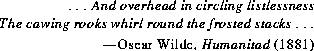# 21.13 The Class `java.util.EmptyStackException`

A `EmptyStackException` is thrown to indicate an attempt to `pop` (§21.12.2) or `peek` (§21.12.3) an empty `Stack` object.

```public class `EmptyStackException` extends RuntimeException {
public `EmptyStackException`();
}
```

21.13.1 `public EmptyStackException()`

This constructor initializes a newly created `EmptyStackException` with `null` as its error message string.

# 21.14 The Class `java.util.NoSuchElementException`

A `NoSuchElementException` is thrown to indicate that another element was requested from an `Enumeration` object that has no more elements to supply. See method `nextElement` of interface `Enumeration` (§21.1.2).

```public class `NoSuchElementException` extends RuntimeException {
public `NoSuchElementException`();
public `NoSuchElementException`(String s);
}
```

21.14.1 `public NoSuchElementException()`

This constructor initializes a newly created `NoSuchElementException` with `null` as its error message string.

21.14.2 `public NoSuchElementException(String s)`

This constructor initializes a newly created `NoSuchElementException` by saving a reference to the error message string `s` for later retrieval by the `getMessage` method (§20.22.3).

Java Language Specification (HTML generated by dkramer on August 01, 1996)